# 二,ESP8266 GPIO和SPI和定时器和串口(基于Lua脚本语言)

+关注继续查看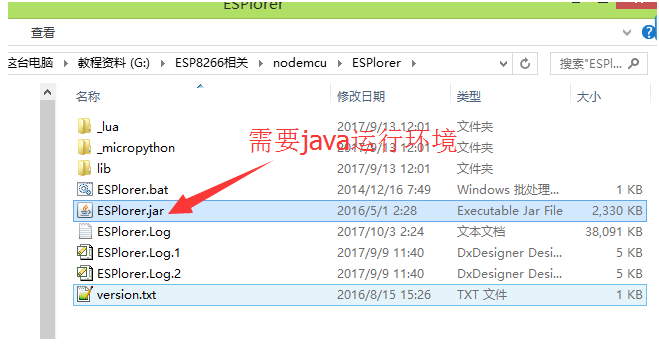http://jingyan.baidu.com/article/3c343ff70bc6ea0d377963df.html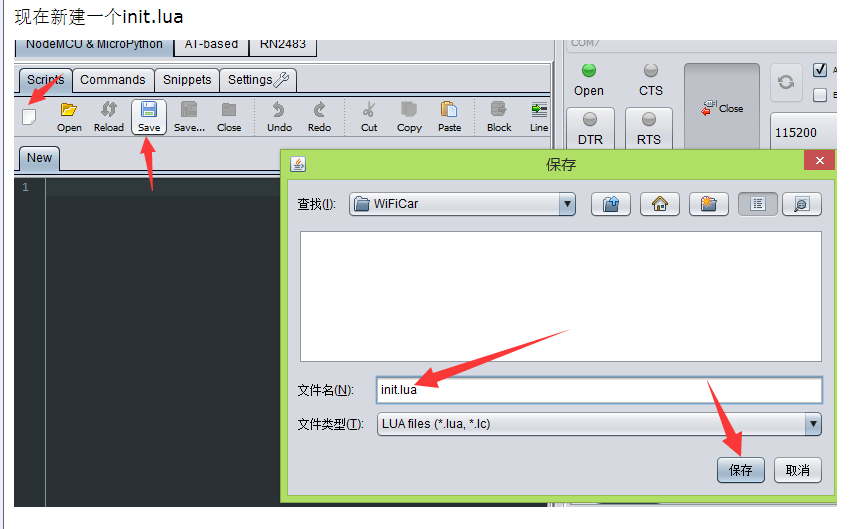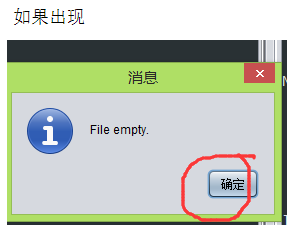让这个灯亮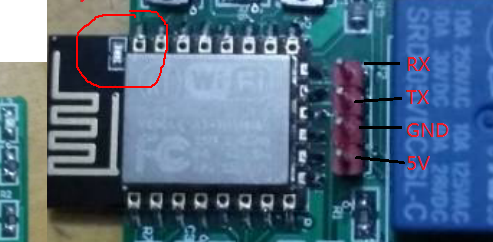gpio.mode(4,gpio.OUTPUT)--输出模式
gpio.write(4,0)--输出低电平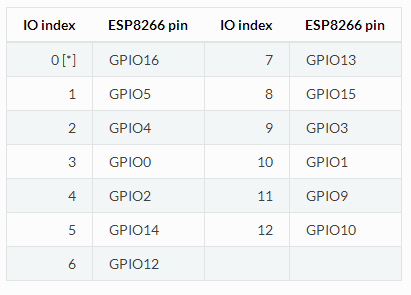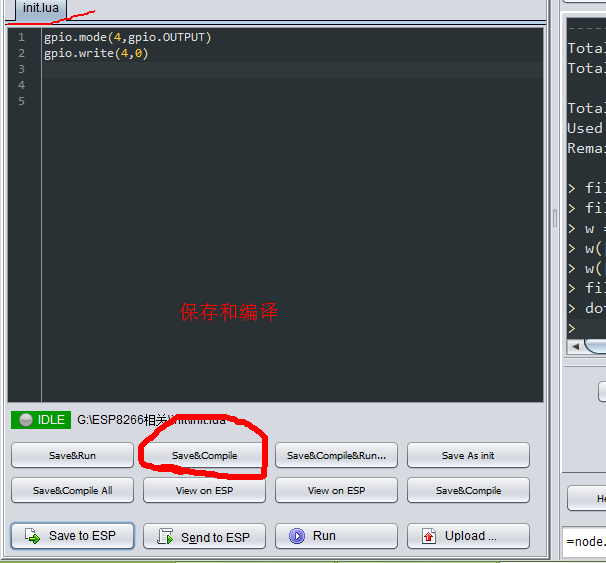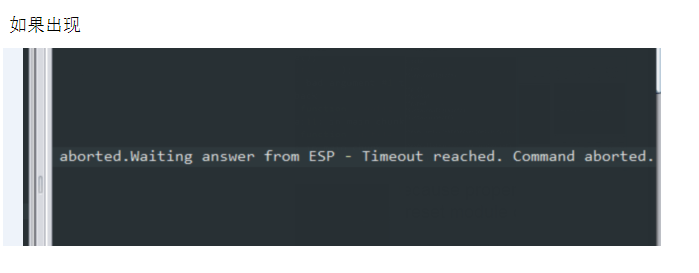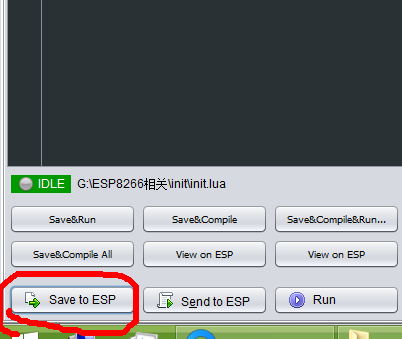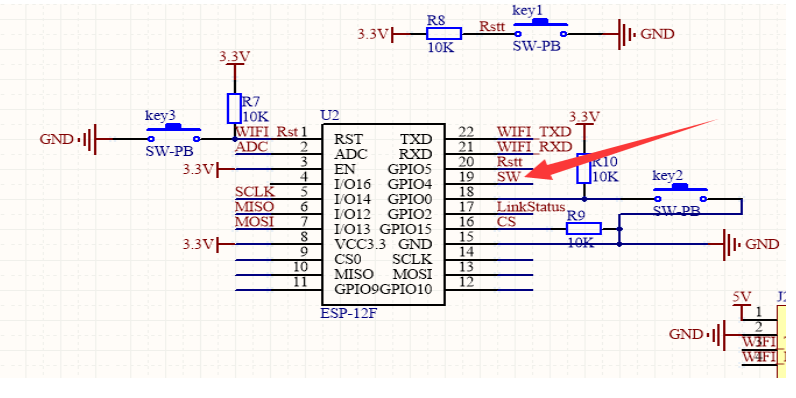gpio.mode(2,gpio.OUTPUT)
gpio.write(2,1)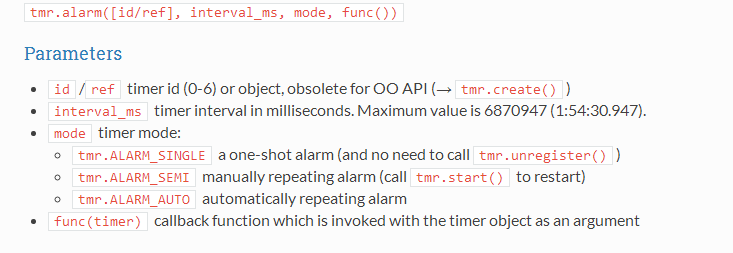[html]
1. gpio.mode(4,gpio.OUTPUT)
2. gpio.write(4,1)
3.
4. function aa()--定义一个函数
5.   print("111111")
6. end
7.
8. tmr.alarm(0, 1000, 1, aa)

0代表用的标号为0的定时器

1000代表延时1000ms执行aa

1代表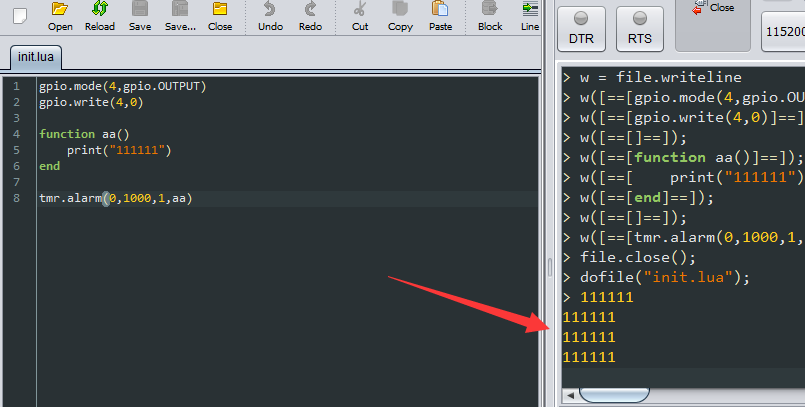[html]
1. gpio.mode(4,gpio.OUTPUT)
2. gpio.write(4,1)
3.
4.
5. tmr.alarm(1, 1000, 1, function()
6.     print("111111")
7. end)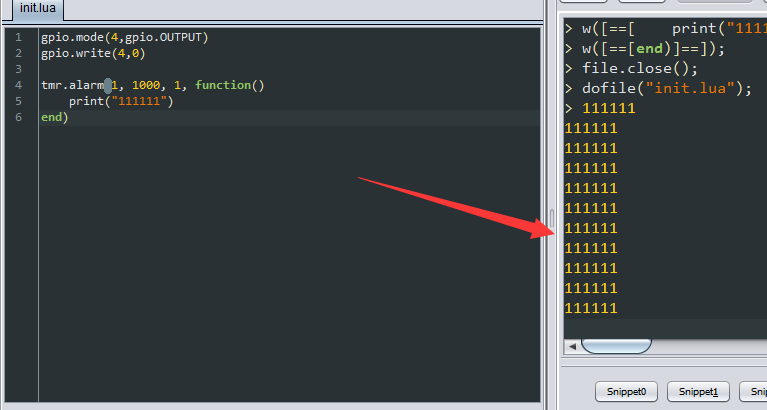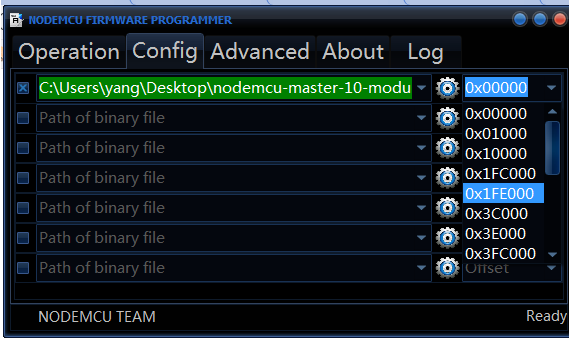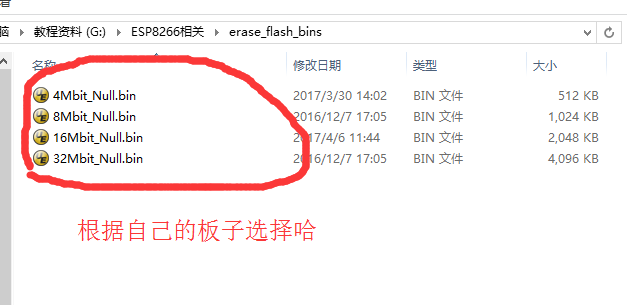http://wiki.ai-thinker.com/_media/esp8266/esp8266_module_list.png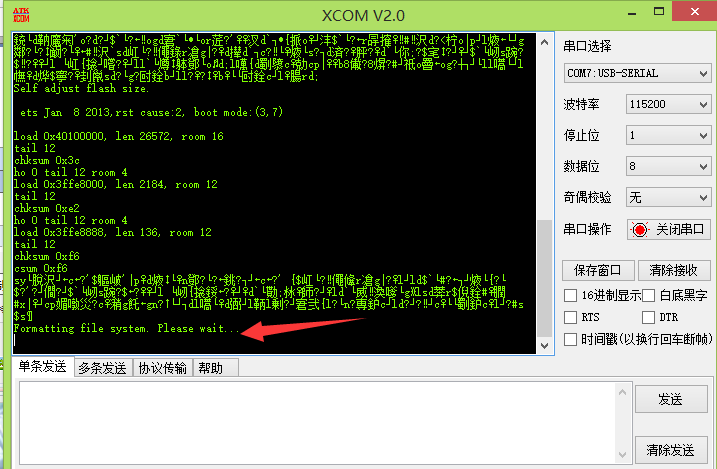等着格式化文件系统,,就等着哈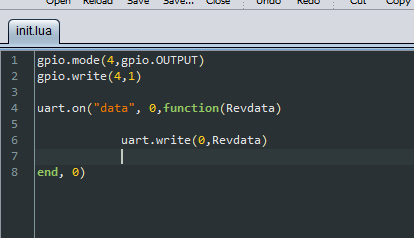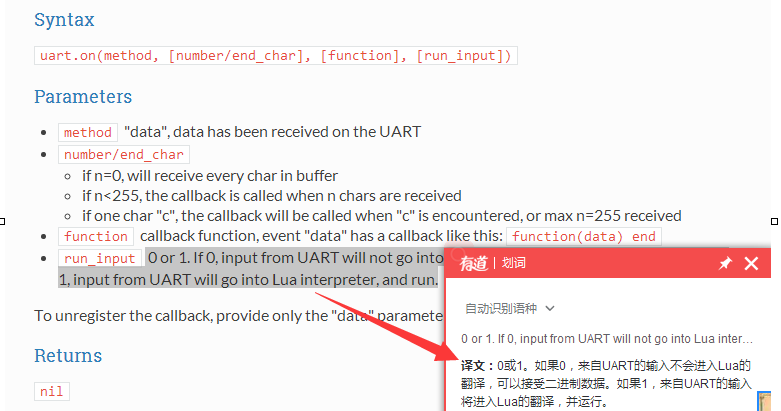gpio.mode(4,gpio.OUTPUT)
gpio.write(4,1)

tmr.alarm(1, 2000, 0, function()

uart.on("data", 0,function(Revdata)

uart.write(0,Revdata)

end, 0)

end)

1.uart.on("data", 0,function(Revdata)
2.
3.            uart.write(0,Revdata)
4.
5.    end, 0)  

uart.write(0,Revdata)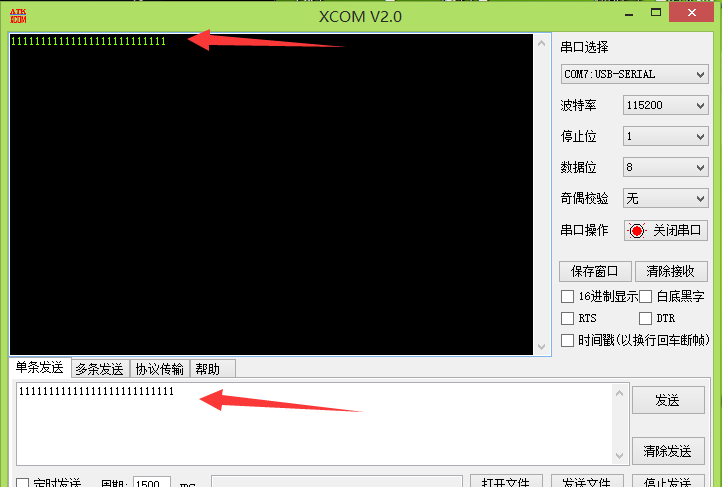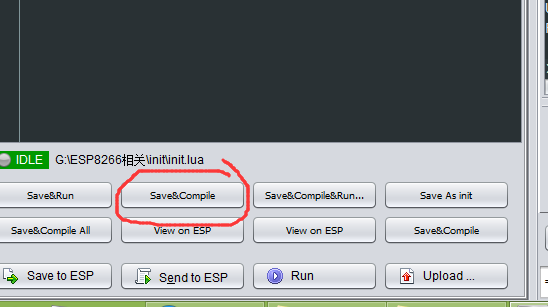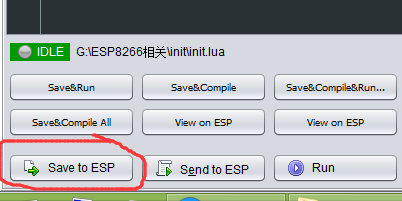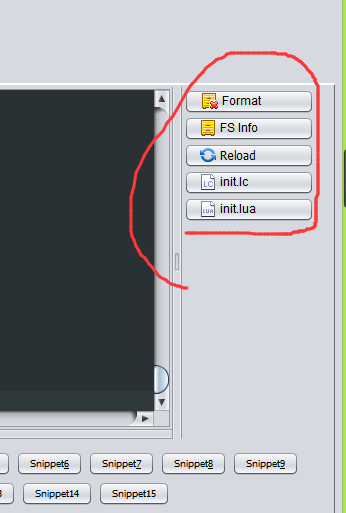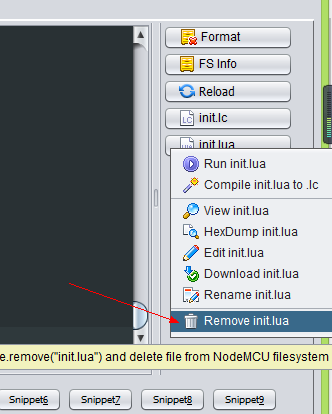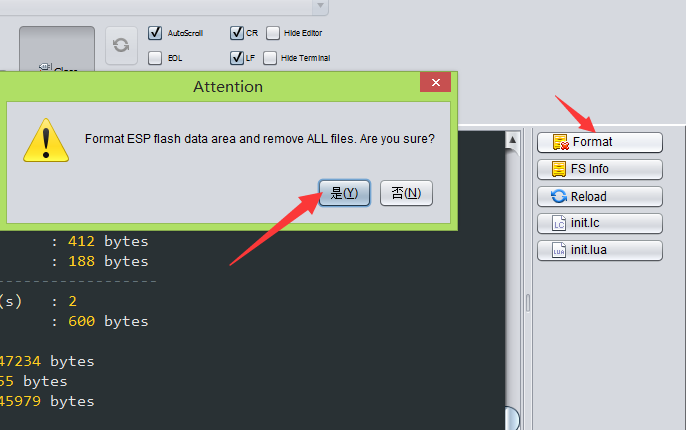print("Relay=1")
print("Relay=0")

[html]
1. gpio.mode(4,gpio.OUTPUT)
2. gpio.mode(2,gpio.OUTPUT)
3. gpio.write(4,1)
4.
5.
6.
7.
8. tmr.alarm(1, 2000, 0, function()
9.
10.
11.     uart.on("data", 0,function(Revdata)
12.
13.
14.             if Revdata == "H" then
15.                gpio.write(2,1)
16.                print("Relay=1")
17.             end
18.
19.             if Revdata == "L" then
20.                gpio.write(2,0)
21.                print("Relay=0")
22.             end
23.
24.             uart.write(0,Revdata)
25.
26.     end, 0)
27.
28. end)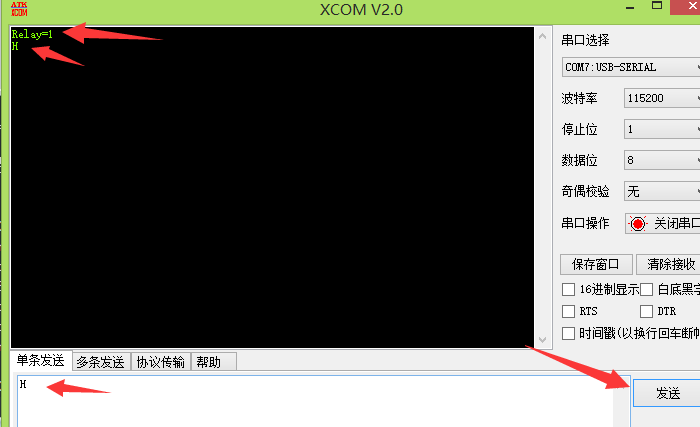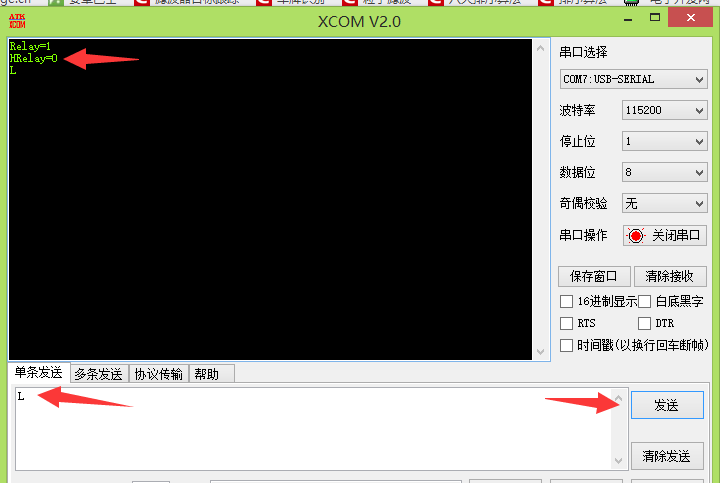[html]
1. gpio.mode(4,gpio.OUTPUT)
2. gpio.mode(2,gpio.OUTPUT)
3. gpio.write(4,1)
4.
5.
6. tmr.alarm(1, 2000, 0, function()
7.
8.     uart.on("data", 0,function(Revdata)
9.
10.             if Revdata == "++H" then
11.                gpio.write(2,1)
12.                print("Relay=1")
13.             end
14.
15.             if Revdata == "++L" then
16.                gpio.write(2,0)
17.                print("Relay=0")
18.             end
19.
20.             uart.write(0,Revdata) -- unregister callback function
21.
22.     end, 0)
23.
24. end)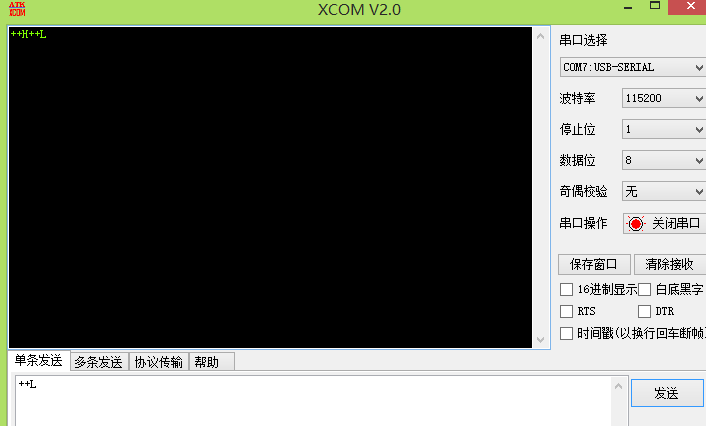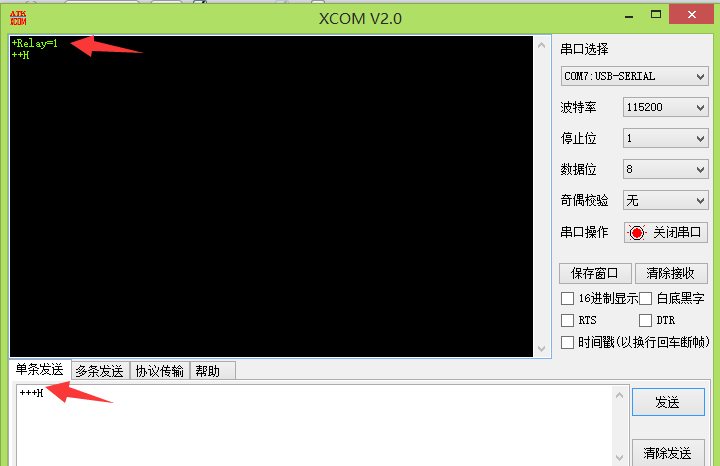[html]
1. gpio.mode(4,gpio.OUTPUT)
2. gpio.mode(2,gpio.OUTPUT)
3. gpio.write(4,1)
4.
5.
6. tmr.alarm(1, 2000, 0, function()
7.
8.     uart.on("data", 0,function(Revdata)
9.
10.             if Revdata == "++H" then
11.                gpio.write(2,1)
12.                print("Relay=1")
13.             else
14.                print(Revdata)----LOOK  LOOK  LOOK
15.             end
16.
17.             if Revdata == "++L" then
18.                gpio.write(2,0)
19.                print("Relay=0")
20.             end
21.
22.             uart.write(0,Revdata) -- unregister callback function
23.
24.     end, 0)
25.
26. end)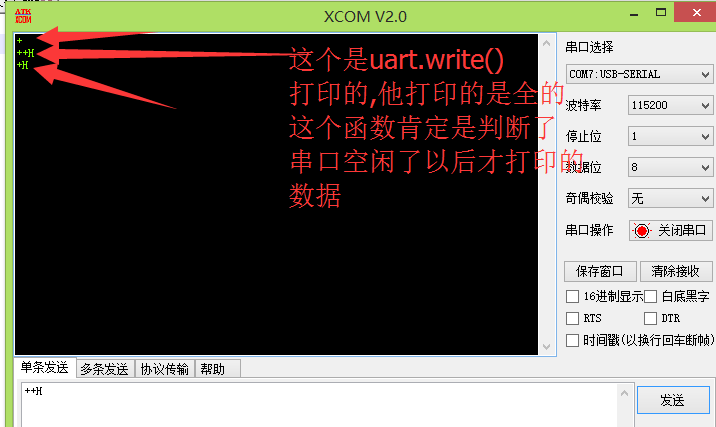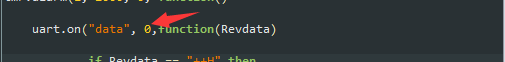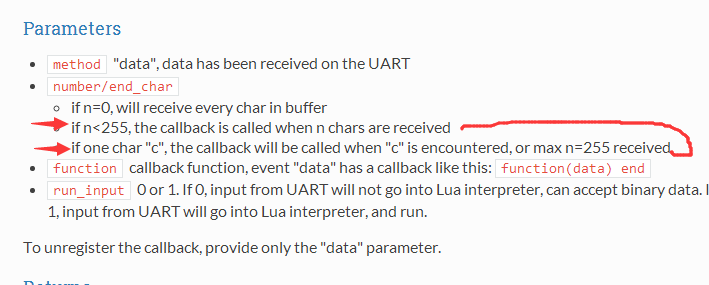[html]
1. gpio.mode(4,gpio.OUTPUT)
2. gpio.mode(2,gpio.OUTPUT)
3. gpio.write(4,1)
4.
5.
10.
11. tmr.alarm(2, 5, 1, function()
12.
13.     if  ReadCnt ~= 0 then
14.
20.
21.             if  ReadDataCopy == "++H" then
22.                 gpio.write(2,1)
23.             end
24.
25.             if  ReadDataCopy == "++L" then
26.                 gpio.write(2,0)
27.             end
28.
29.         else
31.         end
32.
33.     end
34.
35. end)
36.
37.
38.
39. tmr.alarm(1, 2000, 0, function()
40.
41.     uart.on("data", 0,function(Revdata)
42.
45.     end, 0)
46.
47. end)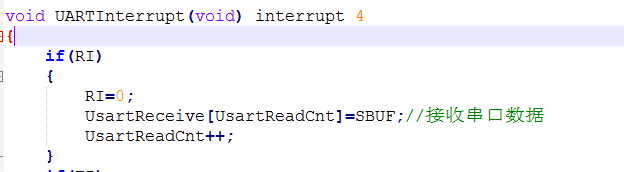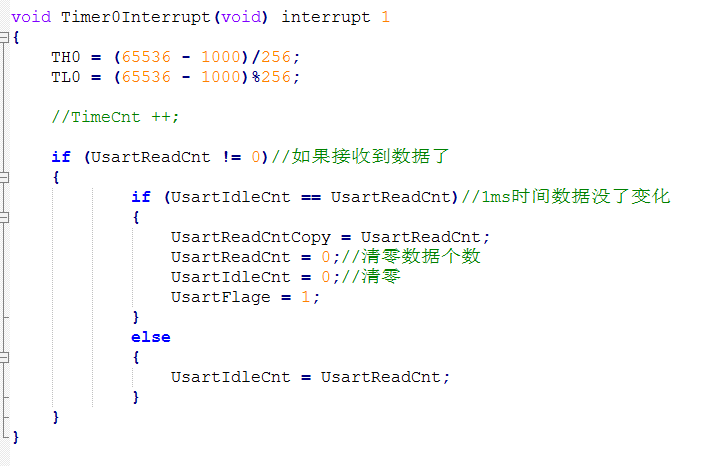crontab脚本，定时任务相关细节

839 018736 0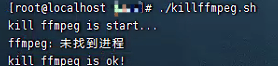Linux定时执行.sh脚本
Linux定时执行.sh脚本
11 023698 0953 01517 0linux定时执行脚本

1128 01107 0linux定时执行脚本
1134 0SQL Server定时自动抓取耗时SQL并归档数据脚本分享

1092 0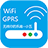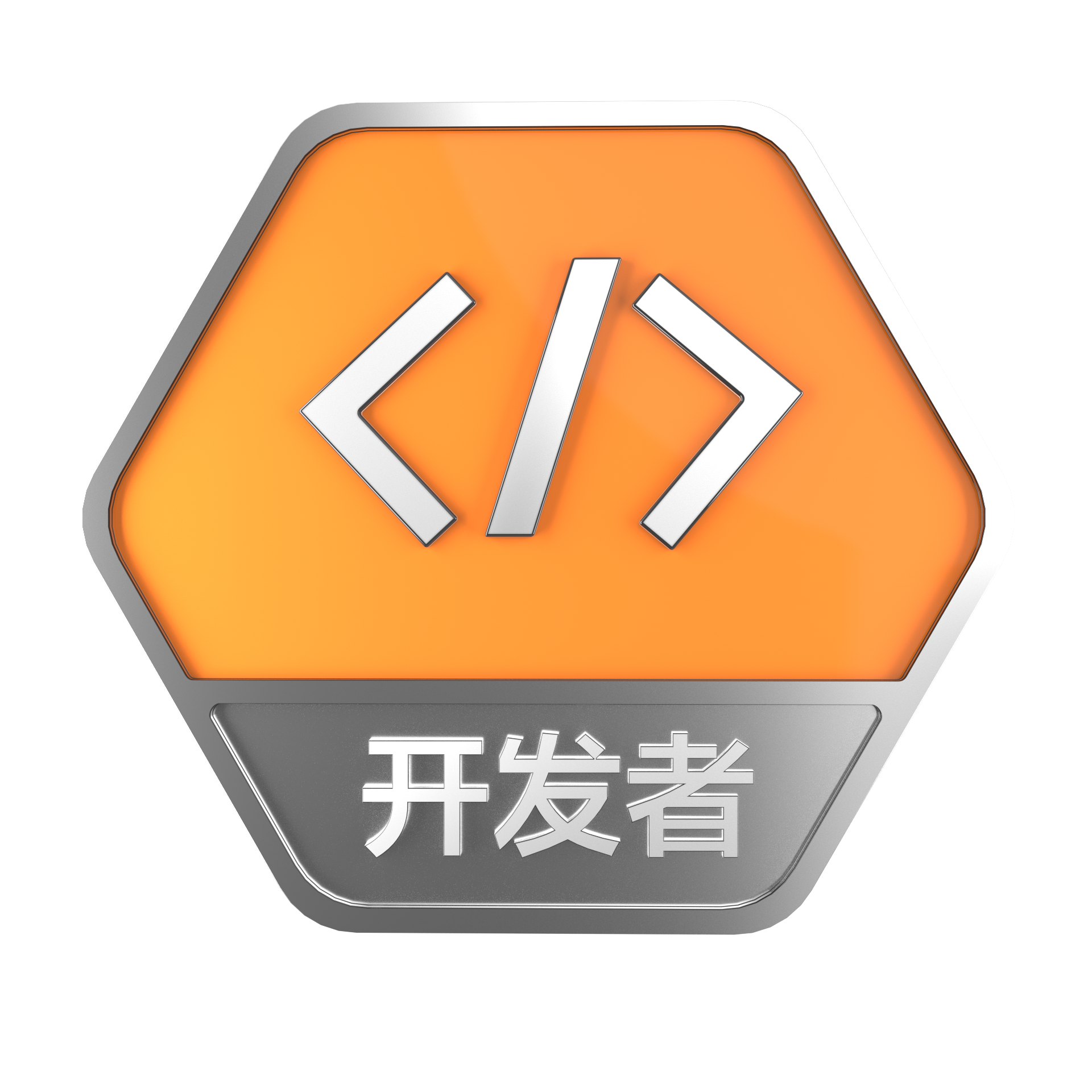+关注

119

0

OceanBase 入门到实战教程